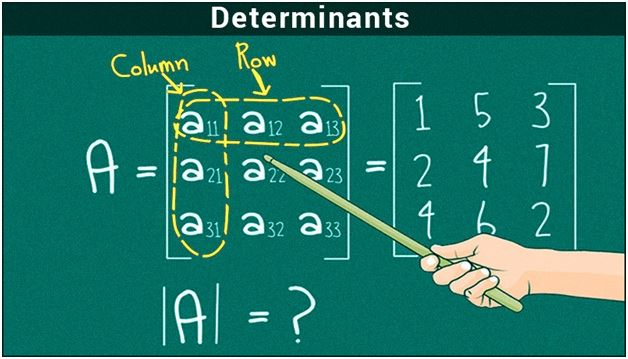# Determinants

A determinant is a word related to algebra, and it is found in most of the calculations in Mathematics. It is implemented in linear equations and also used in the computation of matrices.

## Determinant Meaning

In linear algebra, the determinant is a scalar value that is computed from the elements of the square matrix that obeys certain properties of transformations which are defined by the matrix.As we already know what a matrix is, it is an array of elements or numbers. Hence determinant math of a matrix is defined as the special number or value of a square matrix. Two vertical lines on either side are used to a denote a determinant as shown below-

|X| or det X-This denotes determinant of matrix X.

Suppose X is a matrix with its elements as shown,

$\begin{bmatrix} 3 & 8\\ 4 & 6 \end{bmatrix}$

The determinant of this matrix is |X| calculated as |X| = 6 * 3 – 8 * 4 = |-14|= +14. The determinant is applied to remove the negativity symbol from the values obtained on the calculation of matrices.

## Determinants Properties

Some of the basic properties of determinants as listed below-

• Transpose of a matrix- |Xt|= |X|: This property shows that the determinant for the matrix X is equal to the transpose of a matrix. For example- If |A| = 2 then the transpose matrix for the same will be |At|= -2
• |X|= 0: In this property, the matrix consists of two equal lines having equal values. Hence the resultant matrix would always be zero.
• |A-1| = 1/ A
• |AB|= |A|. |B|: In this property, the det AB is equal to the product of the individual determinants.
• If the elements of any two or two columns are identical, then the determinant value is equal to zero
• If each element of a row or a column is multiplied by the constant, say X, then the value gets multiplied by the constant X
• If any two rows and columns elements of a determinant are interchanged, then the sign of the determinant is also changed

### Determinants Examples

Here you are provided with the examples to solve determinant math of 2 x 2 matrix and 3 x 3 matrix

How to Find the Determinant of 2 * 2 matrix?

Consider a matrix

A= $\begin{bmatrix} a & b\\ c& d \end{bmatrix}$

The det A for this matrix is calculated as follows-

|A| = ad-bc  is the scalar value

For example- if

A= $\begin{bmatrix} 4 & 3\\ 6& 8 \end{bmatrix}$

Then |A| = 4 * 8 – 6 * 3= 32- 18= 14.

How to Find the Determinant of 3 * 3 matrix?

Suppose A is a 3 * 3 matrix consisting of elements-

A= $\begin{bmatrix} a & b & c\\ d& e& f\\ g& h& i \end{bmatrix}$

Then the determinant of matrix A is calculated as

|A| = a(ei – fh) – b(di – fg) + c(dh – eg)

Here, an example is provided to find the determinant of 3 x 3 matrix

A = $\begin{bmatrix} 6 & 1 & 1\\ 4& -2& 5\\ 2& 8& 7 \end{bmatrix}$

The det A is given by |A| = [6×(-2×7 – 5×8) ]- [1×(4×7 – 5×2)] + [1×(4×8 – -2×2)] = [6×(-54)] – [1×(18) ]+ [1×(36)]

|A| = |-306| = 306.

Join BYJU’S and learn more about the applications of determinants, Basics of matrices, Online Maths Formulas and about the different types of Online Maths calculators.